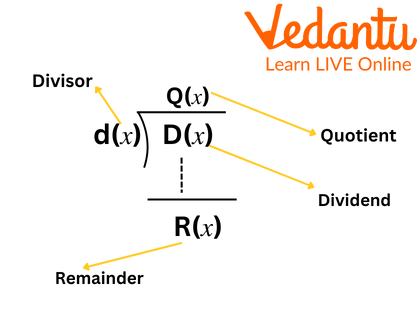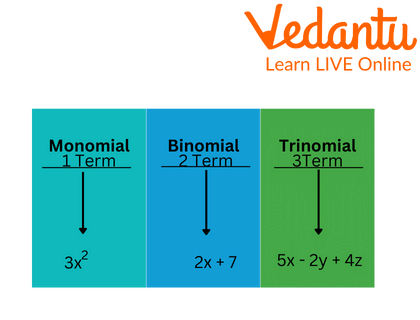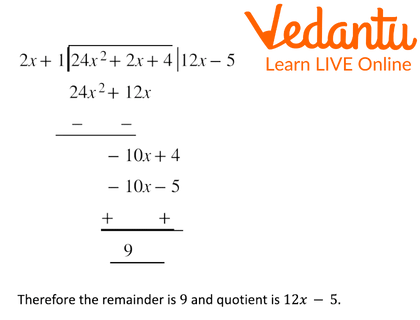Courses
Courses for Kids
Free study material
Free LIVE classes
More

# Long Division Method of PolynomialsLIVE
Join Vedantu’s FREE Mastercalss

## An Overview of the Long Division Method of Polynomials

A generalised version of the well-known arithmetic operation known as long division, polynomial long division is an algorithm for dividing a polynomial by another polynomial of the same or lower degree in algebra. The long division method of polynomials is one of the most common methods of dividing polynomials. In this method, there is a divisor, a dividend (which is to be divided), a quotient, and a reminder. This is a very interesting method of dividing polynomials. The image given below describes the different terms used in the long division method of polynomials.Polynomial Division

## What is a Polynomial?

When exponents, constants and variables are combined using mathematical operations like addition, subtraction, multiplication and division, the result is a polynomial. The kind of the polynomial expression may be monomial, binomial or trinomial, depending on how many terms are included in it.

Example: $9x,2x + 3y,3x + 4z - 9z + 5xyz$ and so on.

## Monomial, Binomial and Trinomial

The number of terms in a polynomial determines which of three different sorts of polynomials it is. There are three different kinds of polynomials:

1. Monomial

2. Binomial

3. TrinomialTypes of Polynomials

### Monomial

Monomial is an expression that contains only one member. There must be only one term which is nonzero. Here are some examples of monomials:

$3x,5y$.

### Binomial

A binomial expression is a polynomial expression containing exactly two terms. A binomial can be thought of as the sum or difference of two or more monomials. Here are some examples of binomials:

$3x + 4y,6a + 5b$.

### Trinomial

A trinomial is an expression with exactly three terms. Here are some examples of trinomial:

$2{x^2} + 3x + 4$

## Long Division Method of Polynomial

Polynomial Division is the process of dividing one polynomial into another. You can perform division between different types of polynomials. Between two monomials, between a polynomial and a monomial, or between two polynomials. A polynomial is an n-algebraic expression with expressive variables, terms and coefficients.

## Steps of Long Division Method of Polynomial

This method of Long Division Polynomials is as follows:

• Sort the terms in descending order of degree.

• Write the missing terms using 0 as the coefficient.

• For the first term of the quotient, divide the first term of the dividend by the first term of the divisor.

• Multiply this quotient by the divisor to get the product.

• Subtract this product from the dividend and omit the next section (if any).

• The difference and the shortened period form a new dividend.

• Follow this process until you get the rest. The remainder can be zero or have an index less than the divisor.Long Division of Polynomials

## Solved Examples on Division Polynomials

1. Solve $\left( {24{x^2} + 2x + 4} \right) \div \left( {2x + 1} \right)$ using a long division method.

Ans: The image below will give a better understanding on how to divide Polynomials.Quotient: 12x - 5

2. How are Polynomials Divided?

Ans: In mathematics, there are two ways to divide polynomials. These are the synthetic approach and lengthy division. The long division method is the most challenging and time-consuming to master, as its name suggests. The synthetic technique, on the other hand, is a "fun" way to divide polynomials.

Last updated date: 26th Sep 2023
Total views: 82.8k
Views today: 0.82k

## FAQs on Long Division Method of Polynomials

1. Write the parts of the division operation.

The parts included in the division operation are divisor, dividend, quotient and remainder.

2. What is a polynomial?

When exponents, constants and variables are combined using mathematical operations like addition, subtraction, multiplication and division, the result is a polynomial.

3. How many types of polynomials are there? Describe briefly.

Polynomial is divided into three parts:

1. Monomial: Polynomial which has one term is known as monomial.

2. Binomial:  Polynomial which has two terms is known as a binomial.

3. Trinomial:  Polynomial which has three terms is known as a trinomial.

4. What is the long division method?

Long division of polynomials is the process of dividing one polynomial into another.

5. Will the remainder always come to zero using the long division method?

No, the remainder can be zero or have an index less than the divisor.